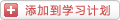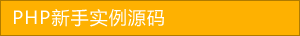﻿ php求两数组交集的三种方法详解 - php数组 - PHP粉丝网

# php求两数组交集的三种方法详解

##### 发布:smiling　来源: PHP粉丝网 　添加日期：2022-06-02 09:50:09　浏览: 评论：0

PHP 代码实现：

1. /**
2.
3.  * @param Integer[] \$nums1
4.
5.  * @param Integer[] \$nums2
6.
7.  * @return Integer[]
8.
9.  */
10.
11. function intersection(\$nums1\$nums2) {
12.
13.     \$res = [];
14.
15.     for(\$i=0;\$i<count(\$nums1);\$i++){
16.
17.         if(in_array(\$nums1[\$i],\$nums2)){
18.
19.             \$res[] = \$nums1[\$i];
20.
21.         }
22.
23.     }
24.
25.     return array_unique(\$res);
26.

1. \$nums2 = [2,4,6,7,8,99];
2.
3. \$nums1 = [1,2,5,9,9,66,89,90,99,99];
4.
5. var_dump(intersection(\$nums1\$nums2));

PHP 代码实现：

1. /**
2.
3.  * @param Integer[] \$nums1
4.
5.  * @param Integer[] \$nums2
6.
7.  * @return Integer[]
8.
9.  */
10.
11. function intersection(\$nums1\$nums2) {
12.
13.     return array_unique(array_intersect(\$nums1,\$nums2));
14.

1. \$nums2 = [2,4,6,7,8,99];
2.
3. \$nums1 = [1,2,5,9,9,66,89,90,99,99];
4.
5. var_dump(intersection(\$nums1\$nums2));

PHP 代码实现：

1. /**
2.
3.  * @param Integer[] \$nums1
4.
5.  * @param Integer[] \$nums2
6.
7.  * @return Integer[]
8.
9.  */
10.
11. function intersection(\$nums1\$nums2) {
12.
13.     \$new_arr = array_merge(array_unique(\$nums1),array_unique(\$nums2));
14.
15.     \$res = [];
16.
17.     for(\$i=0;\$i<count(\$new_arr);\$i++){
18.
19.         for(\$j=\$i+1;\$j<count(\$new_arr);\$j++){
20.
21.             if(\$new_arr[\$i] == \$new_arr[\$j]){
22.
23.                 \$res[] = \$new_arr[\$i];
24.
25.             }
26.
27.         }
28.
29.     }
30.
31.     return array_unique(\$res);
32.

1. \$nums2 = [2,4,6,7,8,99];
2.
3. \$nums1 = [1,2,5,9,9,66,89,90,99,99];
4.
5. var_dump(intersection(\$nums1\$nums2));

PHP 代码实现：

1. /**
2.
3.  * @param Integer[] \$nums1
4.
5.  * @param Integer[] \$nums2
6.
7.  * @return Integer[]
8.
9.  */
10.
11. function intersection(\$nums1\$nums2) {
12.
13.     sort(\$nums1);
14.
15.     sort(\$nums2);
16.
17.     \$i = \$j = 0;
18.
19.     \$res = [];
20.
21.     while(\$i < count(\$nums1) && \$j < count(\$nums2)){
22.
23.         if(\$nums1[\$i] == \$nums2[\$j]){
24.
25.             \$res[] = \$nums1[\$i];
26.
27.             \$i++;
28.
29.             \$j++;
30.
31.         }elseif(\$nums1[\$i] < \$nums2[\$j]){
32.
33.             \$i++;
34.
35.         }elseif(\$nums1[\$i] > \$nums2[\$j]){
36.
37.             \$j++;
38.
39.         }
40.
41.     }
42.
43.     return array_unique(\$res);
44.

1. \$nums2 = [2,4,6,7,8,99];
2.
3. \$nums1 = [1,2,5,9,9,66,89,90,99,99];
4.
5. var_dump(intersection(\$nums1\$nums2));

### 分享到： document.write('<a href="http://v.t.sina.com.cn/share/share.php?url='+encodeURIComponent(location.href)+'&appkey=3172366919&title='+encodeURIComponent('php求两数组交集的三种方法详解')+'" title="分享到新浪微博" class="t1" target="_blank">&nbsp;</a>'); document.write('<a href="http://www.douban.com/recommend/?url='+encodeURIComponent(location.href)+'&title='+encodeURIComponent('php求两数组交集的三种方法详解')+'" title="分享到豆瓣" class="t2" target="_blank">&nbsp;</a>'); document.write('<a href="http://share.renren.com/share/buttonshare.do?link='+encodeURIComponent(location.href)+'&title='+encodeURIComponent('php求两数组交集的三种方法详解')+'" title="分享到人人" class="t3" target="_blank">&nbsp;</a>'); document.write('<a href="http://www.kaixin001.com/repaste/share.php?rtitle='+encodeURIComponent('php求两数组交集的三种方法详解')+'&rurl='+encodeURIComponent(location.href)+'&rcontent=" title="分享到开心网" class="t4" target="_blank">&nbsp;</a>'); document.write('<a href="http://sns.qzone.qq.com/cgi-bin/qzshare/cgi_qzshare_onekey?url='+encodeURIComponent(location.href)+'" title="分享到QQ空间" class="t5" target="_blank">&nbsp;</a>');